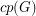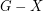Liu, Jiping

Jones' conjecture ★★

Author(s): Kloks; Lee; Liu

For a graph, letdenote the cardinality of a maximum cycle packing (collection of vertex disjoint cycles) and letdenote the cardinality of a minimum feedback vertex set (set of verticesso thatis acyclic).

Conjecture   For every planar graph,.

Keywords: cycle packing; feedback vertex set; planar graph